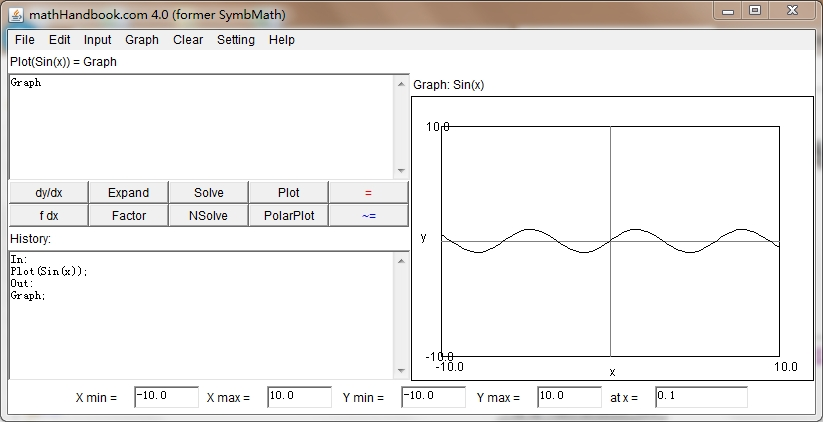﻿ ﻿﻿ Fractional Calculus Computer Algebra System math software Home | list | math | function | calculator | handbook | help | forum | contact | index | 中文﻿
﻿

lasolve

solve differential and fractional differential equation for y and system of equations for x(t) and y(t) by laplace transform.

Calling Sequence Parameters Description Examples References Source

Calling Sequence

lasolve(eq)
lasolve(eq, y)
lasolve(right side of eq, y,x)
lasolve(right side of eq, y,x,n)
lasolve(eq 1, eq 2)

Parameters

eq - equation.
y - default unknown y(x).
x - variable x.
n - the nth order of equation.

Description

For first order differential equation, there are 3 ways to input derivative:
1. y'
2. y(1,x)
3. ds(y) or ds(y,x)
where ds() is a derivative holder.

For second order differential equation, there are 3 ways to input derivative:
1. y''
2. y(2,x)
3. ds(y,x,2)
where y'' is two sinlge quote sign, instead one double quote sign.

For fractional order differential equation, there are 2 ways to input derivative:
1. y(0.5,x)
2. ds(y,x,0.5)

Solution can be tested by odetest(). If odetest(solution,eq) give 0, solution satisfy the equation.

Example

Input first order equation:
> y(1,x)=2y
> y'=2y
> ds(y)=2y
Click the lasolve button to call lasolve():
> lasolve(y(1,x)=2y)
> lasolve(y'=2y)
> lasolve(ds(y)=2y)
> lasolve(2y,y,x)

Input second order equation:
> y(2,x)=2y
> ds(y,x,2)=2y
> y''=2y
> lasolve(ds(y,x,2)=2y)
> lasolve(y''=2y)
> lasolve(2y,y,x,2)

Input fractional 0.5 order equation:
> ds(y,x,0.5)=2y
> lasolve(ds(y,x,0.5)=2y)
> lasolve(2y,y,x,0.5)

References

solve, dsolve, rsolve, nsolve, ﻿